Fifth Mississippi State Conference on Differential Equations and Computational Simulations,
Electron. J. Diff. Eqns., Conf. 10, 2003, pp. 153-161.

### A selfadjoint hyperbolic boundary-value problem Nezam Iraniparast

Abstract:
We consider the eigenvalue wave equation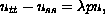subject to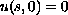, where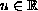, is a function of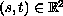, with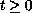. In the characteristic triangle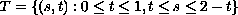we impose a boundary condition along characteristics so that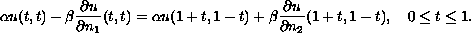The parametersand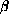are arbitrary except for the condition that they are not both zero. The two vectors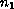and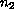are the exterior unit normals to the characteristic boundaries and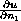,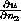are the normal derivatives in those directions. When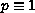we will show that the above characteristic boundary value problem has real, discrete eigenvalues and corresponding eigenfunctions that are complete and orthogonal in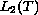. We will also investigate the case where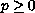is an arbitrary continuous function in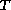.

Published February 28, 2003.
Subject classifications: 35L05, 35L20, 35P99.
Key words: Characteristics, eigenvalues, eigenfunctions, Green's function, Fredholm alternative.

Show me the PDF file (189K), TEX file, and other files for this article.Nezam Iraniparast Department of Mathematics Western Kentucky University E-mail: nezam.iraniparast@wku.edu